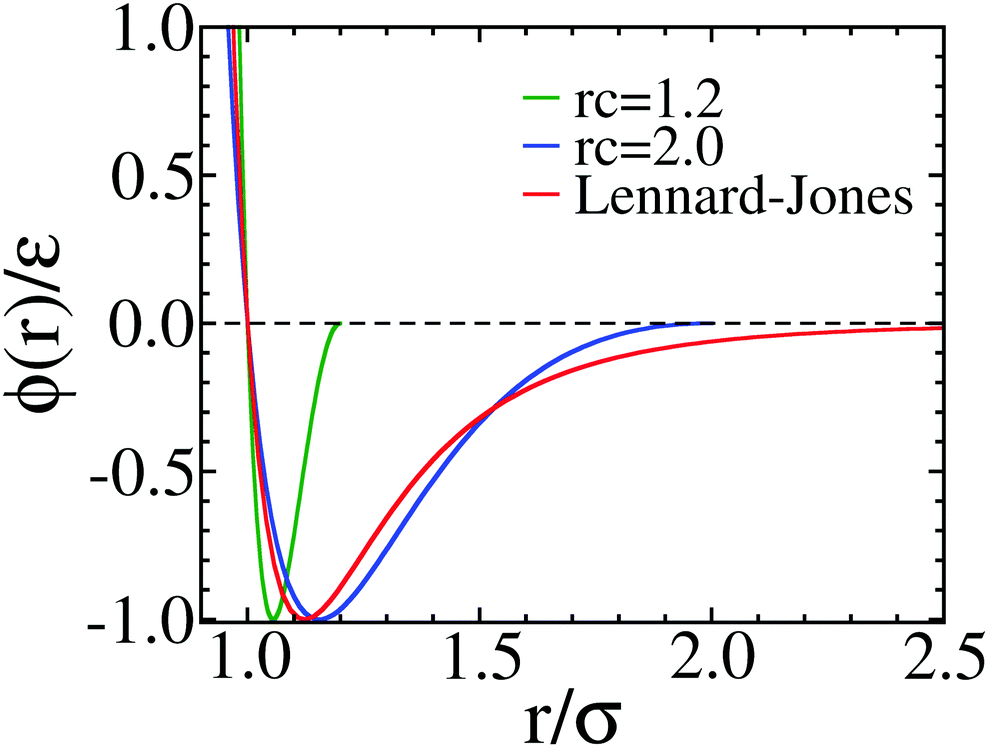# pair_style wf/cut command¶

## Syntax¶

pair_style wf/cut cutoff

• cutoff = cutoff for wf interactions (distance units)

## Examples¶

pair_style        wf/cut 2.0
pair_coeff        1 1 1.0 1.0 1 1  2.0


## Description¶

The wf/cut (Wang-Frenkel) style computes LJ-like potentials as described in Wang2020. This potential is by construction finite ranged and it vanishes quadratically at the cutoff distance, avoiding truncation, shifting, interpolation and other typical procedures with the LJ potential. The wf/cut can be used when a typical short-ranged potential with attraction is required. The potential is given by which is given by:

$\phi(r)= \epsilon \alpha \left(\left[{\sigma\over r}\right]^{2\mu} -1 \right)\left(\left[{r_c\over r}\right]^{2\mu}-1\right)^{2\nu}$

with

$\alpha=2\nu\left(\frac{r_c}{\sigma}\right)^{2\mu}\left[\frac{1+2\nu}{2\nu\left[(r_c/\sigma)^{2\mu}-1\right]}\right]^{2\nu+1}$

and

$r_{min}=r_c\left[\frac{1+2\nu}{1+2\nu(r_c/\sigma)^{2\nu}}\right]^{1/{2\nu}}$

$$r_c$$ is the cutoff.

Comparison of the non-truncated Lennard-Jones 12-6 potential (red curve), and the WF potentials with $$\mu=1$$ and $$\nu=1$$ are shown in the figure below. The blue curve has $$r_c =2.0$$ and the green curve has $$r_c =1.2$$ and can be used to describe colloidal interactions.The following coefficients must be defined for each pair of atoms types via the pair_coeff command as in the example above, or in the data file or restart files read by the read_data or read_restart commands:

• $$\epsilon$$ (energy units)

• $$\sigma$$ (distance units)

• $$\nu$$

• $$\mu$$

• $$r_c$$ (distance units)

The last coefficient is optional. If not specified, the global cutoff given in the pair_style command is used. The exponents $$\nu$$ and $$\mu$$ are positive integers, usually set to 1. There is usually little to be gained by choosing other values of $$\nu$$ and $$\mu$$ (See discussion in Wang2020)

Mixing, shift, table, tail correction, restart, rRESPA info:

This pair style does not support the pair_modify mixing and table options.

The pair_modify tail option is not relevant for this pair style as it goes to zero at the cut-off radius.

This pair style writes its information to binary restart files, so pair_style and pair_coeff commands do not need to be specified in an input script that reads a restart file.

This pair style does not support the use of the inner, middle, and outer keywords of the run_style respa command.

## Restrictions¶

This pair style can only be used if LAMMPS was built with the EXTRA-PAIR package. See the Build package doc page for more info.